### First Law of Thermodynamics

The first law of thermodynamics has been first proposed by MAYER2.8 in 1841 : Heat is a kind of energy and can therefore neither be created nor destroyed.'' Hence, heat has been defined as the transfered thermal energy between two systems if they are brought into thermal contact . The infinitesimal change of the internal energy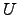of a system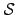can be expressed as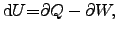(2.28)

where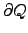is the infinitesimal heat added to the system and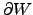represents the infinitesimal work performed by the system. This equation follows directly from the total differential of the entropy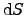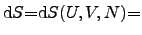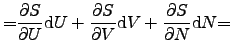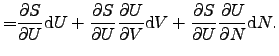(2.29)

With the equations (2.22)-(2.24), the total differential of the entropy becomes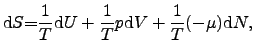(2.30)

where the pressure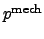can be found as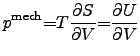(2.31)

and the chemical potential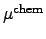as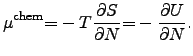(2.32)

By comparison of the coefficients from (2.28) and (2.30)-(2.32), the net heat flow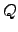and the work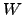done by the systemcan be found as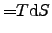(2.33)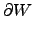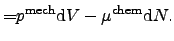(2.34)

Stefan Holzer 2007-11-19